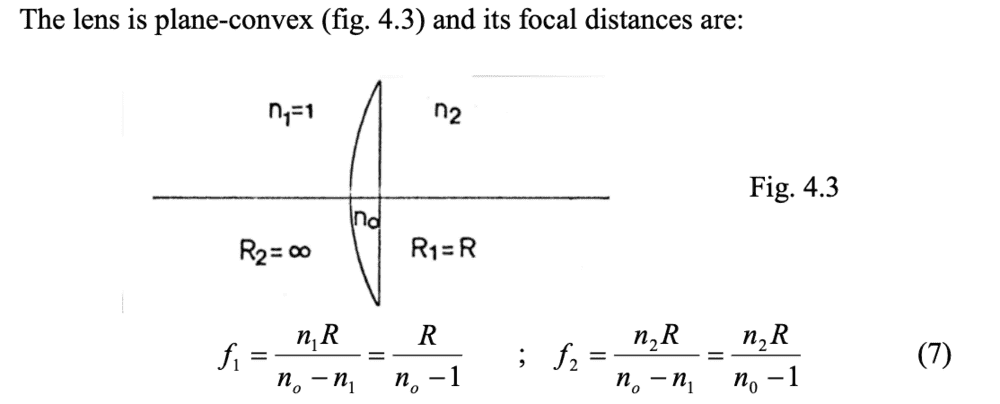# Lensmaker's equation when the 2 mediums on both sides of the lens are different

phantomvommand
Hi, I chanced upon an interesting diagram lately, and was wondering what the lensmakers equation for such a lens would be. Attached below is the diagram. Basically, the refractive indexes of the 2 mediums surrounding each side of the lens are different.

They have also calculated the focal distances as shown below. However, I notice some inconsistency. f1 seems not to involve the refractive index of the opposite medium, while f2 does. Is the calculation of focal length correct, and what is the lensmakers equation when the mediums around the lens are different?Last edited by a moderator: